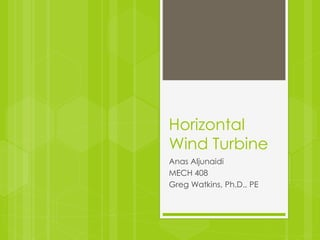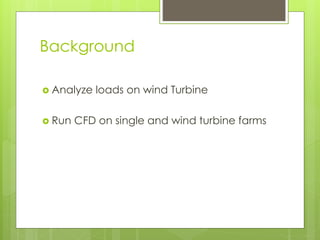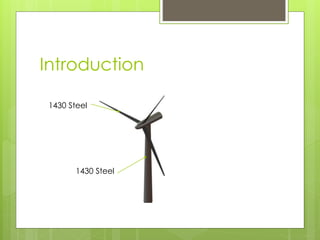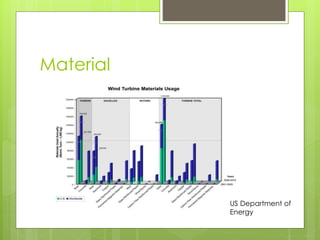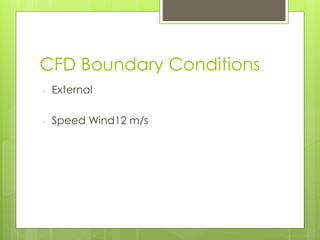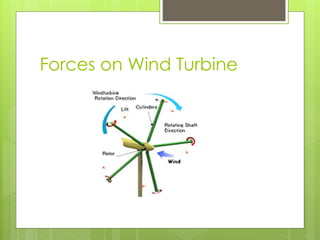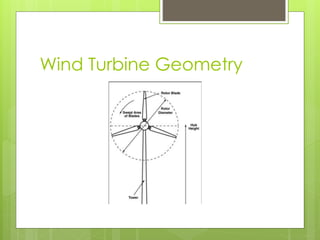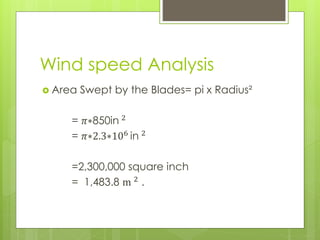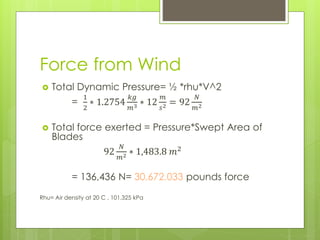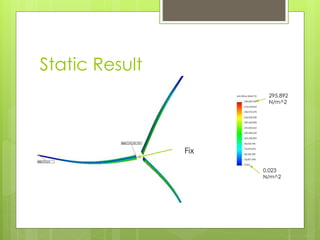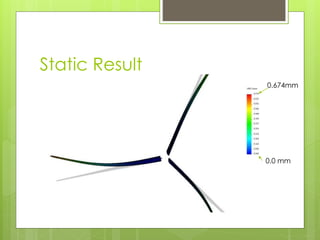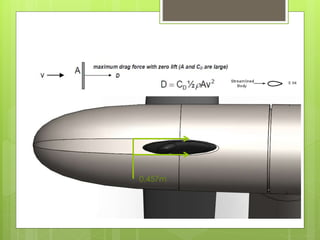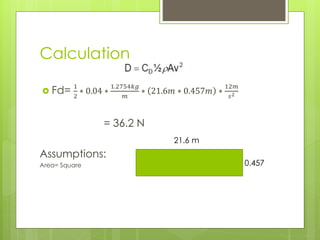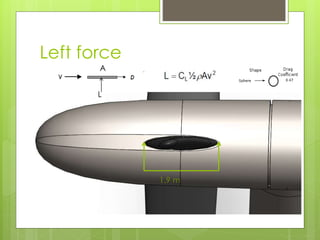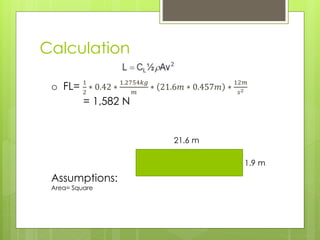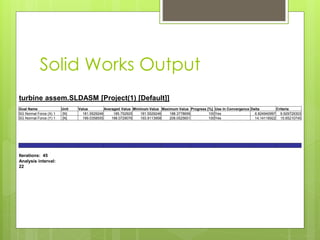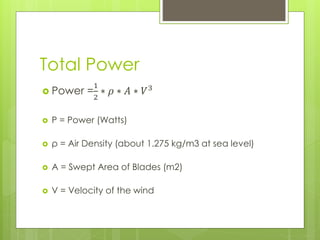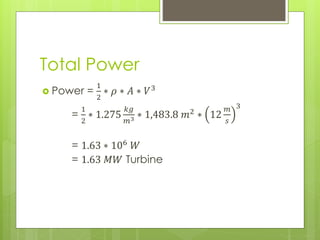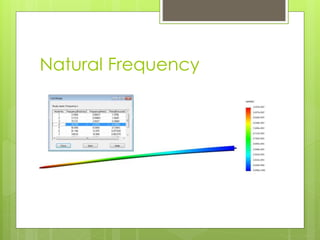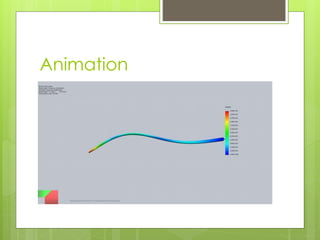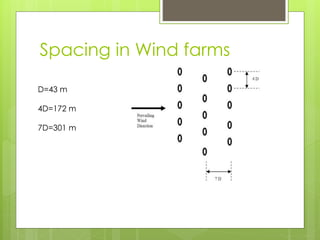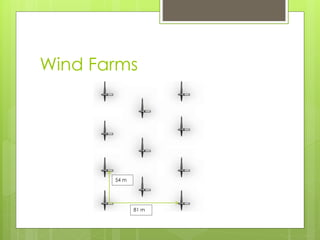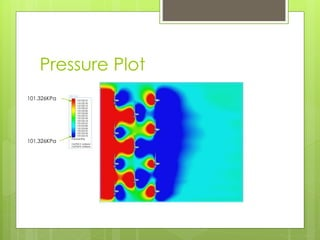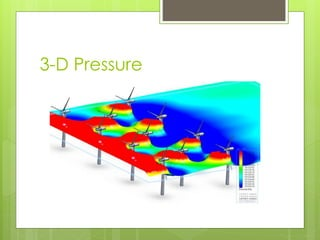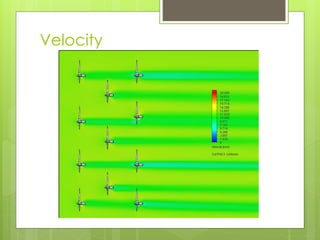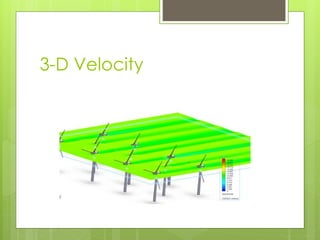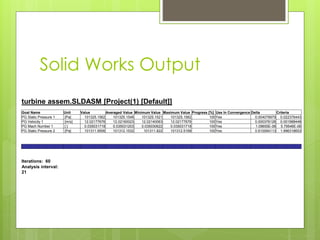1 de 27

### Horizontal Wind Turbine

1. Horizontal Wind Turbine Anas Aljunaidi MECH 408 Greg Watkins, Ph.D., PE
2. Background  Analyze loads on wind Turbine  Run CFD on single and wind turbine farms
3. Introduction 1430 Steel 1430 Steel
4. Material US Department of Energy
5. CFD Boundary Conditions - External - Speed Wind12 m/s
6. Forces on Wind Turbine
7. Wind Turbine Geometry
8. Wind speed Analysis  Area Swept by the Blades= pi x Radius² = 𝜋∗850in 2 = 𝜋∗2.3∗106 in 2 =2,300,000 square inch = 1,483.8 m 2 .
9. Force from Wind  Total Dynamic Pressure= ½ *rhu*V^2 = 1 2 ∗ 1.2754 𝑘𝑔 𝑚3 ∗ 12 𝑚 𝑠2 = 92 𝑁 𝑚2  Total force exerted = Pressure*Swept Area of Blades 92 𝑁 𝑚2 ∗ 1,483.8 𝑚2 = 136,436 N= 30,672.033 pounds force Rhu= Air density at 20 C , 101.325 kPa
10. Static Result Fix 0.023 N/m^2 295,892 N/m^2
11. Static Result 0.674mm 0.0 mm
12. Drag 0.457m
13. Calculation  Fd= 1 2 ∗ 0.04 ∗ 1.2754𝑘𝑔 𝑚 ∗ 21.6𝑚 ∗ 0.457𝑚 ∗ 12𝑚 𝑠2 = 36.2 N Assumptions: Area= Square 21.6 m 0.457
14. Left force 1.9 m L A
15. Calculation o FL= 1 2 ∗ 0.42 ∗ 1.2754𝑘𝑔 𝑚 ∗ 21.6𝑚 ∗ 0.457𝑚 ∗ 12𝑚 𝑠2 = 1,582 N Assumptions: Area= Square 21.6 m 1.9 m
16. Solid Works Output turbine assem.SLDASM [Project(1) [Default]] Goal Name Unit Value Averaged Value Minimum Value Maximum Value Progress [%] Use In Convergence Delta Criteria SG Normal Force (X) 1 [N] 181.5529246 185.752925 181.5529246 188.3778656 100Yes 6.824940997 9.929729303 SG Normal Force (Y) 1 [N] 199.0358555 198.0729076 193.9113958 208.0525651 100Yes 14.14116922 15.65210745 Iterations: 45 Analysis interval: 22
17. Total Power  Power = 1 2 ∗ 𝜌 ∗ 𝐴 ∗ 𝑉3  P = Power (Watts)  ρ = Air Density (about 1.275 kg/m3 at sea level)  A = Swept Area of Blades (m2)  V = Velocity of the wind
18. Total Power  Power = 1 2 ∗ 𝜌 ∗ 𝐴 ∗ 𝑉3 = 1 2 ∗ 1.275 𝑘𝑔 𝑚3 ∗ 1,483.8 𝑚2 ∗ 12 𝑚 𝑠 3 = 1.63 ∗ 106 𝑊 = 1.63 𝑀𝑊 Turbine
19. Natural Frequency
20. Animation
21. Spacing in Wind farms D=43 m 4D=172 m 7D=301 m
22. Wind Farms 81 m 54 m
23. Pressure Plot 101.326KPa 101.326KPa
24. 3-D Pressure
25. Velocity
26. 3-D Velocity
27. Solid Works Output turbine assem.SLDASM [Project(1) [Default]] Goal Name Unit Value Averaged Value Minimum Value Maximum Value Progress [%] Use In Convergence Delta Criteria PG Static Pressure 1 [Pa] 101325.1562 101325.1548 101325.1521 101325.1562 100 Yes 0.004078979 0.022376443 PG Velocity 1 [m/s] 12.02177676 12.02160023 12.02140063 12.02177676 100 Yes 0.000376126 0.001988448 PG Mach Number 1 [ ] 0.035031718 0.035031203 0.035030622 0.035031718 100 Yes 1.09655E-06 5.79546E-06 PG Static Pressure 2 [Pa] 101311.9506 101312.1532 101311.822 101312.5168 100 Yes 0.610094113 1.996318653 Iterations: 60 Analysis interval: 21